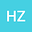Periodic solutions of a second-order iterative differential equation
•• Houyu Zhao
Houyu Zhao
Chongqing Normal University

Corresponding Author:houyu19@gmail.com

Author Profile## Abstract

In this paper, we use Schauder and Banach fixed point theorem to study the existence, uniqueness and stability of periodic solutions of a class of iterative differential equation $$\alpha x’‘(t)+\beta x’(t)+\gamma x(t)=\lambda_1(t)x(t)+\lambda_2(t)x(x(t))+\cdots+\lambda_n(t)x^{[n]}(t)+f(t).$$